# sklearn.metrics.accuracy_score¶

sklearn.metrics.accuracy_score(y_true, y_pred, normalize=True, sample_weight=None)[source]

Accuracy classification score.

In multilabel classification, this function computes subset accuracy: the set of labels predicted for a sample must exactly match the corresponding set of labels in y_true.

Read more in the User Guide.

Parameters: y_true : 1d array-like, or label indicator array / sparse matrix Ground truth (correct) labels. y_pred : 1d array-like, or label indicator array / sparse matrix Predicted labels, as returned by a classifier. normalize : bool, optional (default=True) If False, return the number of correctly classified samples. Otherwise, return the fraction of correctly classified samples. sample_weight : array-like of shape = [n_samples], optional Sample weights. score : float If normalize == True, return the fraction of correctly classified samples (float), else returns the number of correctly classified samples (int). The best performance is 1 with normalize == True and the number of samples with normalize == False.

Notes

In binary and multiclass classification, this function is equal to the jaccard_similarity_score function.

Examples

>>> import numpy as np
>>> from sklearn.metrics import accuracy_score
>>> y_pred = [0, 2, 1, 3]
>>> y_true = [0, 1, 2, 3]
>>> accuracy_score(y_true, y_pred)
0.5
>>> accuracy_score(y_true, y_pred, normalize=False)
2


In the multilabel case with binary label indicators:

>>> accuracy_score(np.array([[0, 1], [1, 1]]), np.ones((2, 2)))
0.5


## Examples using sklearn.metrics.accuracy_score¶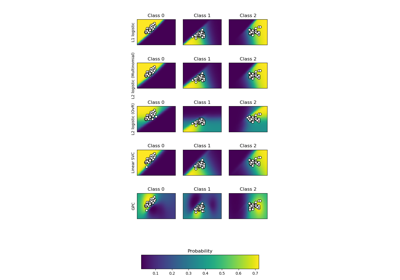Plot classification probability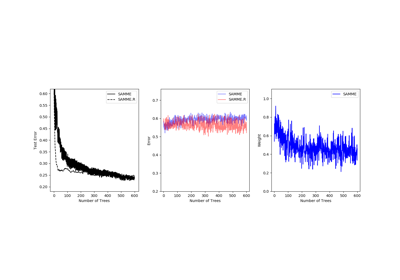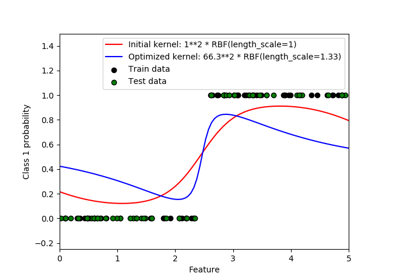Probabilistic predictions with Gaussian process classification (GPC)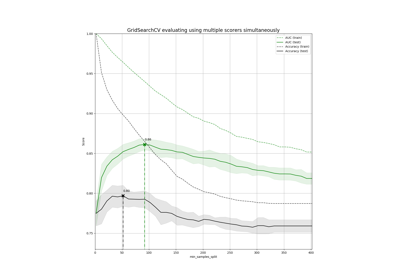Demonstration of multi-metric evaluation on cross_val_score and GridSearchCV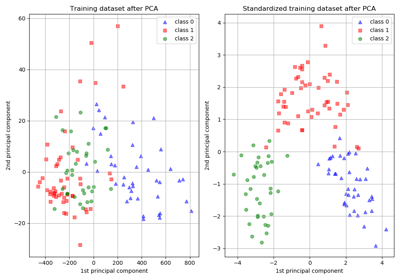Importance of Feature Scaling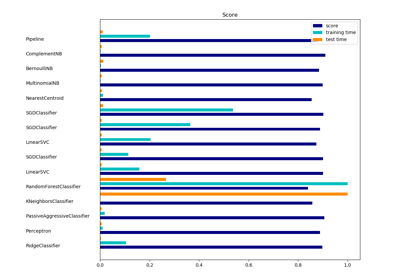Classification of text documents using sparse features